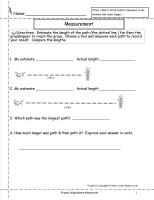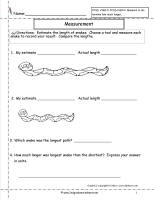﻿ CCSS 2.MD.4 Worksheets, Measure and Estimate Worksheets

# Common Core State Standard 2.MD.4

## Measurement & Data

### Measure and estimate lengths in standard units.

Measure to determine how much longer one object is than another, expressing the length difference in terms of a standard length unit.

###Measure and Compare Lengths

Common Core State Standards: 2.MD.4
Measurement & Data
Measure and estimate lengths in standard units.

Measure to determine how much longer one object is than another, expressing the length difference in terms of a standard length unit.

Students measure two objects and compare the lengths.

###Measure and Compare Lengths

Common Core State Standards: 2.MD.4
Measurement & Data
Measure and estimate lengths in standard units.

Measure to determine how much longer one object is than another, expressing the length difference in terms of a standard length unit.

Students measure two objects and compare the lengths.